# Subgradient optimization: Difference between revisions

This web page is a duplicate of https://optimization.mccormick.northwestern.edu/index.php/Subgradient_optimization

Author Name: Aaron Anderson (ChE 345 Spring 2015)
Steward: Dajun Yue and Fengqi You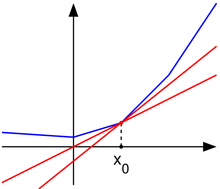A convex nondifferentiable function (blue) with red "subtangent" lines generalizing the derivative at the nondifferentiable point x0.

Subgradient Optimization (or Subgradient Method) is an iterative algorithm for minimizing convex functions, used predominantly in Nondifferentiable optimization for functions that are convex but nondifferentiable. It is often slower than Newton's Method when applied to convex differentiable functions, but can be used on convex nondifferentiable functions where Newton's Method will not converge. It was first developed by Naum Z. Shor in the Soviet Union in the 1960's.

## Introduction

The Subgradient (related to Subderivative and Subdifferential) of a function is a way of generalizing or approximating the derivative of a convex function at nondifferentiable points. The definition of a subgradient is as follows: $g$is a subgradient of $f$at $x$if, for all $y$, the following is true:

An example of the subgradient of a nondifferentiable convex function $f$can be seen below:

Where $g_{1}$is a subgradient at point $x_{1}$and $g_{2}$and $g_{3}$are subgradients at point $x_{2}$. Notice that when the function is differentiable, such as at point $x_{1}$, the subgradient, $g_{1}$, just becomes the gradient to the function. Other important factors of the subgradient to note are that the subgradient gives a linear global underestimator of $f$and if $f$is convex, then there is at least one subgradient at every point in its domain. The set of all subgradients at a certain point is called the subdifferential, and is written as $\partial f(x_{0})$at point $x_{0}$.

Suppose $f:\mathbb {R} ^{n}\to \mathbb {R}$is a convex function with domain $\mathbb {R} ^{n}$. To minimize $f$the subgradient method uses the iteration:

Where $k$is the number of iterations, $x^{(k)}$is the $k$th iterate, $g^{(x)}$is any subgradient at $x^{(k)}$, and $\alpha _{k}$$(>0)$is the $k$th step size. Thus, at each iteration of the subgradient method, we take a step in the direction of a negative subgradient. As explained above, when $f$is differentiable, $g^{(k)}$simply reduces to $\nabla$$f(x^{(k)})$. It is also important to note that the subgradient method is not a descent method in that the new iterate is not always the best iterate. Thus we need some way to keep track of the best solution found so far, i.e. the one with the smallest function value. We can do this by, after each step, setting

and setting $i_{\text{best}}^{(k)}=k$if $x^{(k)}$is the best (smallest) point found so far. Thus we have:

which gives the best objective value found in $k$iterations. Since this value is decreasing, it has a limit (which can be $-\infty$).

An algorithm flowchart is provided below for the subgradient method:

### Step size

Several different step size rules can be used: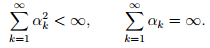One typical example is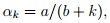where $a>0$and $b\geq 0$.
• Nonsummable diminishing: These step sizes satisfy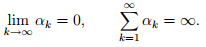One typical example is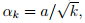where $a>0$.

An important thing to note is that for all four of the rules given here, the step sizes are determined "off-line", or before the method is iterated. Thus the step sizes do not depend on preceding iterations. This "off-line" property of subgradient methods differs from the "on-line" step size rules used for descent methods for differentiable functions where the step sizes do depend on preceding iterations.

### Convergence Results

There are different results on convergence for the subgradient method depending on the different step size rules applied. For constant step size rules and constant step length rules the subgradient method is guaranteed to converge within some range of the optimal value. Thus:

where $f^{*}$is the optimal solution to the problem and $\epsilon$is the aforementioned range of convergence. This means that the subgradient method finds a point within $\epsilon$of the optimal solution $f^{*}$. $\epsilon$is number that is a function of the step size parameter $h$, and as $h$decreases the range of convergence $\epsilon$also decreases, i.e. the solution of the subgradient method gets closer to $f^{*}$with a smaller step size parameter $h$. For the diminishing step size rule and the square summable but not summable rule, the algorithm is guaranteed to converge to the optimal value or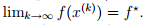When the function $f$is differentiable the subgradient method with constant step size yields convergence to the optimal value, provided the parameter $h$is small enough.

## Example: Piecewise linear minimization

Suppose we wanted to minimize the following piecewise linear convex function using the subgradient method:

Since this is a linear programming problem finding a subgradient is simple: given $x$we can find an index $j$for which:

The subgradient in this case is $g=a_{j}$. Thus the iterative update is then:

Where $j$is chosen such to satisfy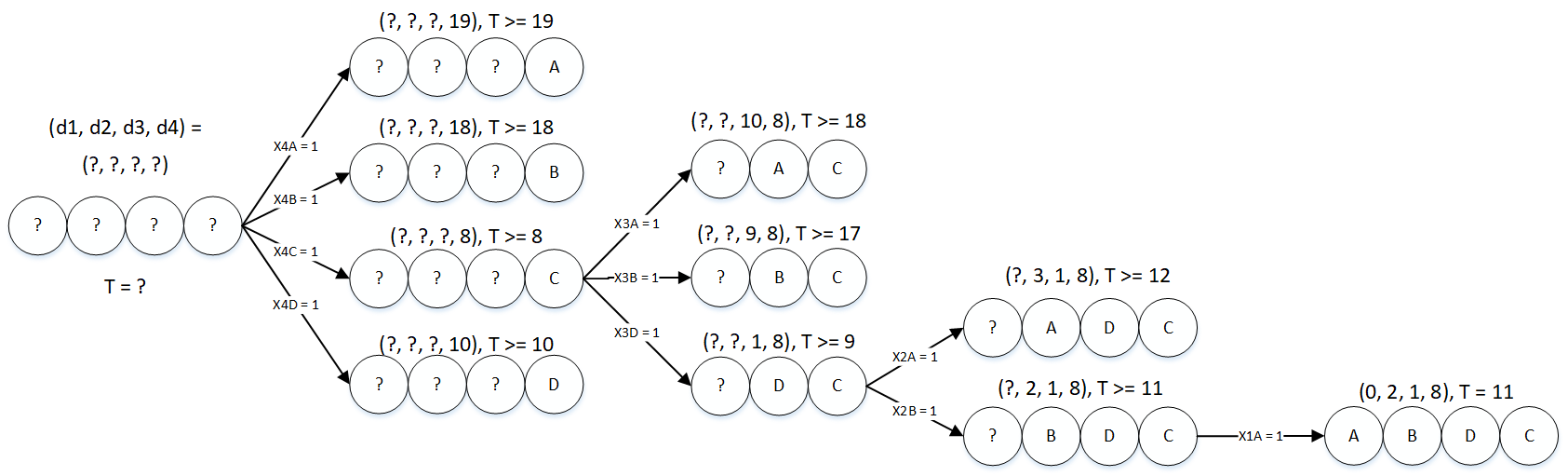In order to apply the subgradient method to this problem all that is needed is some way to calculate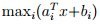and the ability to carry out the iterative update. Even if the problem is dense and very large (where standard linear programming might fail), if there is some efficient way to calculate $f$then the subgradient method is a reasonable choice for algorithm. Consider a problem with $n=10$variables and $m=100$terms and with data $a_{i}$and $b_{i}$generated from a normal distribution. We will consider all four of the step size rules mentioned above and will plot $\epsilon$or the difference between the optimal solution and the subgradient solution as a function of $k$, the nuber of iterations.
For the constant step size rule File:Example6.png for several values of $h$the following plot was obtained: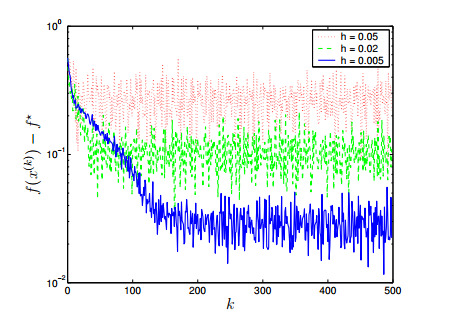For the constant step length rule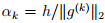for several values of $h$the following plot was obtained: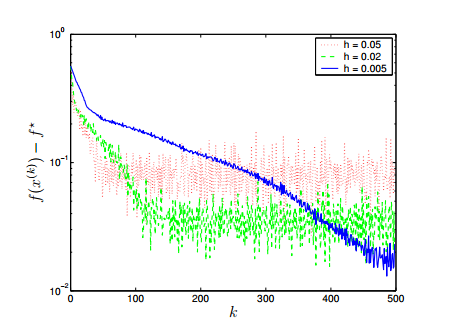The above figures reveal a trade-off: a larger step size parameter $h$gives a faster convergence but in the end gives a larger range of suboptimality so it is important to determine an $h$that will converge close to the optimal solution without taking a very large number of iterations.
For the subgradient method using diminishing step size rules, both the nonsummable diminishing step size rule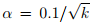(blue) and the square summable but not summable step size rule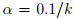(red) are plotted below for convergence: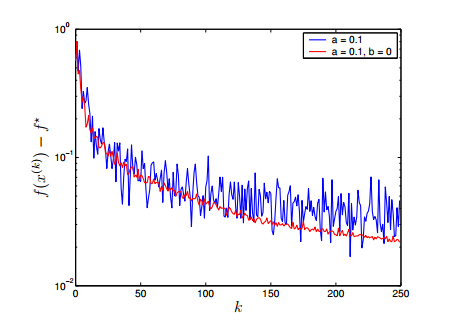This figure illustrates that both the nonsummable diminishing step size rule and the square summable but not summable step size rule show relatively fast and good convergence. The square summable but not summable step size rule shows less variation than the nonsummable diminishing step size rule but both show similar speed and convergence.
Overall, all four step size rules can be used to get good convergence, so it is important to try different values for $h$in the constant step size and length rules and different formulas for the nonsummable diminishing step size rule and the square summable but not summable step size rule in order to get good convergence in the smallest amount of iterations possible.

## Conclusion

The subgradient method is a very simple algorithm for minimizing convex nondifferentiable functions where newton's method and simple linear programming will not work. While the subgradient method has a disadvantage in that it can be much slower than interior-point methods such as Newton's method, it as the advantage of the memory requirement being often times much smaller than those of an interior-point or Newton method, which means it can be used for extremely large problems for which interior-point or Newton methods cannot be used. Morever, by combining the subgradient method with primal or dual decomposition techniques, it is sometimes possible to develop a simple distributed algorithm for a problem. The subgradient method is therefor an important method to know about for solving convex minimization problems that are nondifferentiable or very large.